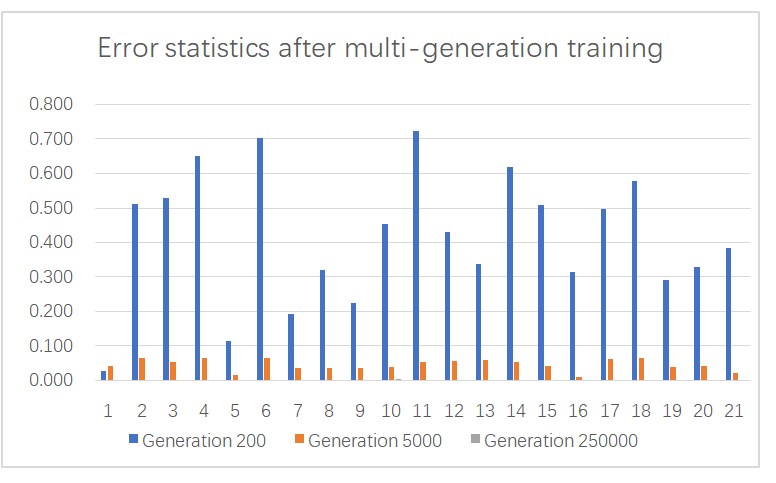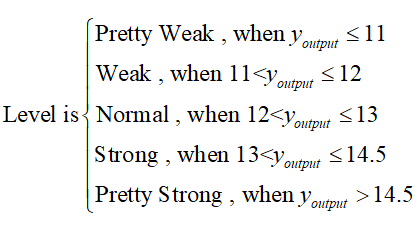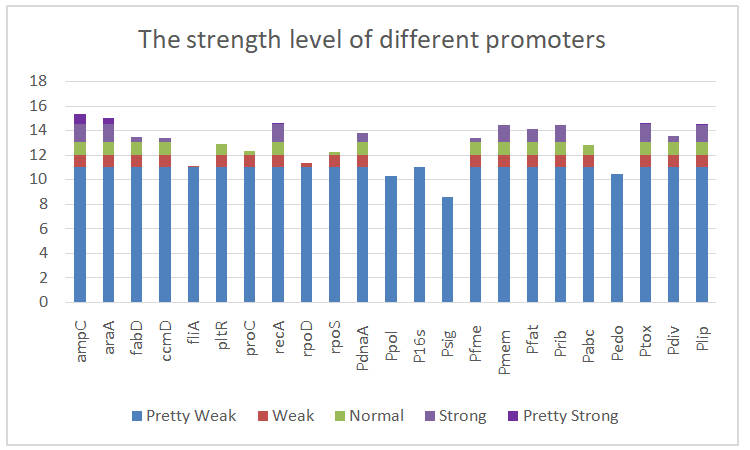# Team:SKLMT-China/Model Results

## results

Through the test of the linear relationship of sparse equations, we prove that there is a strong linear correlation between the promoter strength and the number of different triple bases, and strengthen this linear relationship with the mode of deep learning. We call the process of using all known data in turn for a round of learning a generation.The fitting results of the model under different generations are given below:It can be seen that the more generations we calculate, the better the data fitting degree we can get, and the absolute error is even lower than 0.01 after the 250, 000 repetitions of training.This indicates that the data fitting precision is very high.

After setting the number of learning rounds as 250, 000 generations, the coefficients between neurons in the input layer, hidden layer and output layer were obtained respectively. The complete table was placed in the appendix part.As long as a core sequence of DNA is input, our model can obtain the output value of promoter strength immediately with the existing parameters.The specific calculation process is as follows:

$$y_{output}=\sum_{i=1}^{6}y_{i}\cdot p_{i}\, ,\, y_{i}=\sum_{j=1}^{64}\omega _{ji}x_{j}$$

We notice that there is a significant difference in the magnitude of the measurements between our group and the other groups. In order to better contribute our results to other teams, we will use the magnitude of our measurements and classify the promoters by numerical size.

The promoter intensity level corresponding to the data is as follows:Using this standard, we quickly categorized 23 known promoters:With the expansion of the promoter data, we can quickly calculate the strength level of the new promoter with the help of our model.

## Further application

In order to be of greater help to other teams, we would like to attach the source code of all the programs in the appendix for you to read and verify. At the same time, we also designed python programs to enable our research results to interact well with other teams.

1. Provide convenient data insertion for other teams. When a new set of data is measured, just submit the core sequence and measurement values through our interface (please take the natural logarithm of the original data first), and then the data will be entered into our database.

2. Easily control the speed and accuracy of deep learning by changing the learning generation. After a new generation is set, the program can automatically update all parameters with the new generation.

3.Predict promoter strength. After entering the core sequence, our python program will calculate the strength level of the promoter for you.